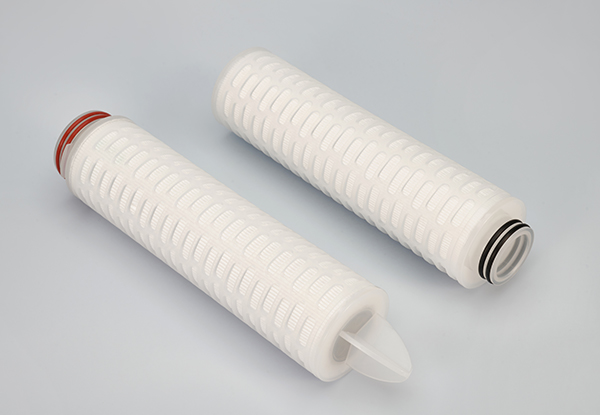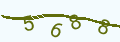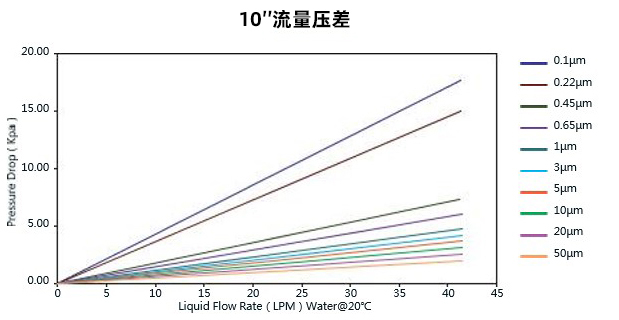## PP滤芯

GVS的PP折叠滤芯由超细纤维聚丙烯膜和纯PP支撑层折叠而成，有效扩大了滤芯使用面积，独特工艺赋予高纳污能力和高通量的特性，滤芯整体构造均由聚丙烯制成，生产制作采用热熔技术，不含任何粘合剂，使用安全，广泛应用于各种领域。

### 信息咨询/样品申请

#### 信息类型

• 密理博
• 赛多利斯
• 颇尔
• 赛默飞
• 其他
• 了解
• 不了解
• 百度搜索
• 微信平台
• 展会
• 代理商
• 第三方平台● 过滤面积大，流量与纳污量高

RO前置保安过滤外径 69mm 长度 5”,10”,20”,30”,40” 滤材 聚丙烯（PP） 导流层材质 聚丙烯（PP） 外护网/支撑层/端盖材质 聚丙烯（PP） 密封圈 硅橡胶（三元乙丙橡胶、 氟橡胶、特氟隆橡胶可选） 过滤面积 0.5 m2/10”滤芯 工作温度高达 80°C 最大工作压差 正向 4.0 Bar @ 20℃

CFPPP 0010=0.1μm 5 = 5" G=226带卡翅/尖头 E=EPDM P=聚丙烯

0020=0.2μm 10=10" H=226带卡翅/平头 S=Silicone

0045=0.45μm 20=20" I=226不带卡翅 / 平头 V=Viton

0100=1μm 30=30" J=222带卡翅 / 尖头 T=Teflon

0300=3μm 40=40" K=222不带卡翅 / 尖头

0500=5μm   L=222不带卡翅 / 平头

1000=10μm   M=DOE接口

2000=20μm

5000=50μm